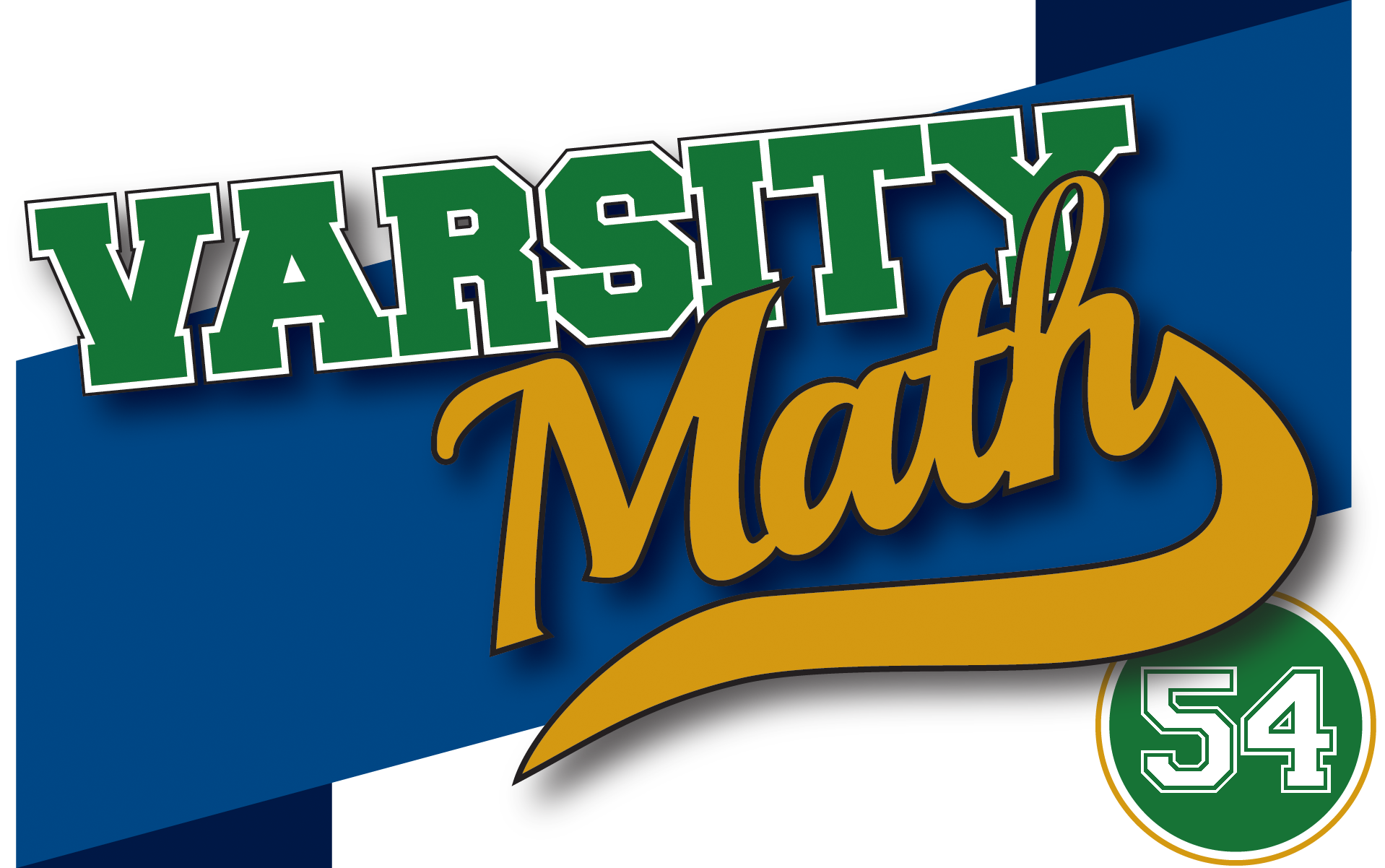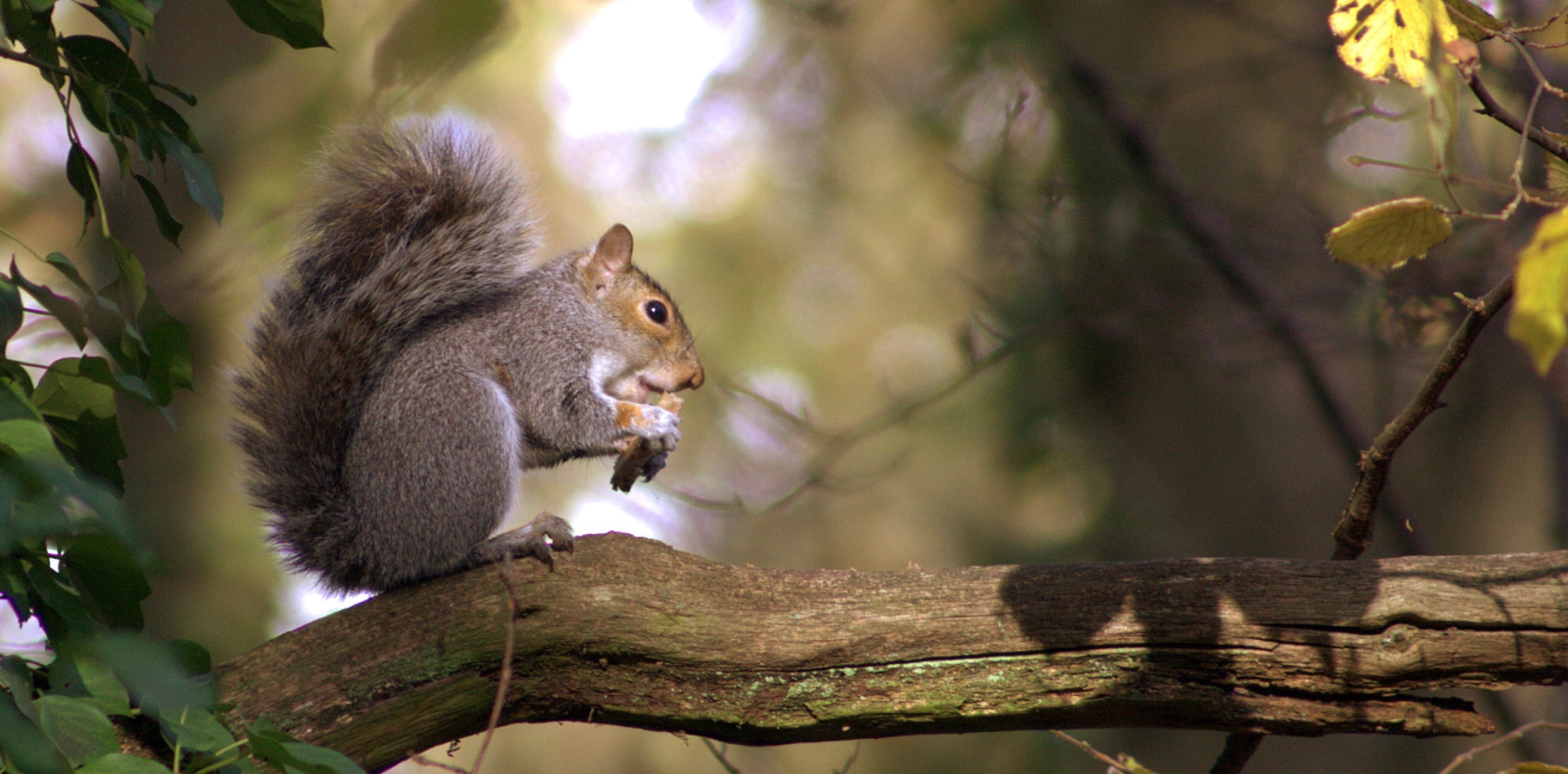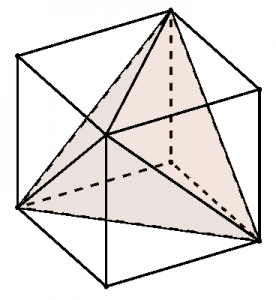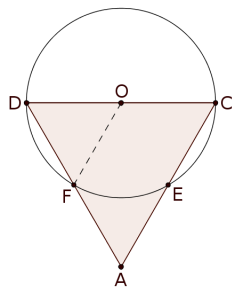## ________________

In honor of the first week of fall, the Varsity Math team is working on problems about things that fall.

## ________________### Watch for Falling Nuts

Two squirrels knock acorns off a tree with identical horizontal velocity (and initially no vertical velocity, although the constant acceleration due to gravity quickly propels both acorns toward the ground, which happens to be perfectly level in the vicinity of this tree). The first squirrel knocks its acorn off from six meters above the ground. The other acorn lands twice as far away from the tree as the first one. Assume that air resistance has no effect on the motion of the acorns.

From how high did the second squirrel knock off its acorn?

### Fall Out of Line

If you set up a row of dominoes on end with appropriate spacing between them (as shown from a bird’s-eye view in the diagram) and then push over the domino at one end (A) it will set off a chain reaction resulting in the domino at the other end (B) falling. Conversely, if you push B over, it will cause A to fall.How can you arrange dominoes, leaving A and B where they are, but possibly rearranging the others and/or adding more dominoes if needed, so that pushing A over will make B fall, but not vice versa?

## Solutions to week 53

Hexadiagonal.The six diagonals all meet up and have no “loose ends” and form a pyramid when they only connect to four non-adjacent vertices of the cube, out of the eight vertices overall, as in the diagram. (It doesn’t matter which of the two possible sets of four vertices you use, you get an equivalent solid.) You can see that the resulting pyramid, shaded in the diagram, contains the center of the cube and can be formed by chopping off four identical right-triangular pyramids, each of which has one of the “unused” vertices at its apex. If we let the edge of the cube be our unit (since we are just looking for a fraction of the cube’s volume anyway), then each of the pyramids to chop off has a base area equal to one half, and a height of one, and so has a volume of 1/6 of the cube (1/3 of base times height). Since we need to chop four of them off, we have eliminated 4/6 of the cube’s volume, so the remaining pyramid has a volume of 2/6 = 1/3 of the cube’s volume.

Hexintersection.Since the radius of the sphere is half of the edge length of the tetrahedron and its center is at the midpoint of an edge, two of the points of intersection of the sphere and the edges of the tetrahedron are two vertices of the tetrahedron, labeled C and D in the diagram at left. You can also see in the diagram that there are four more points of intersection, one on each of the other edges that connect to C or D. But how do we know where the points of intersection are located along those edges? Figuring that out for point F (for example) is much easier if we just look in the plane of the face ADC.In that plane, the cross section of the sphere is a circle with the same center O and diameter DC. Since segment OF is the same length as OD, triangle FOD is equilateral, and hence FD also has length 3/2 (see the small diagram). In other words, F is the midpoint of tetrahedron edge AD, and the same goes for the other three intersection points, E, G, and the unlabeled point H at the back of the sphere. But that means that all of the edge lengths and angles of solid ABEFGH are the same as those of DCEFGH, so they are congruent solids, and hence each must have 1/2 the volume of the original tetrahedron.

## Recent Weeks

Links to all of the puzzles and solutions are on the Complete Varsity Math page.

Come back next week for answers and more puzzles.

[asciimathsf]